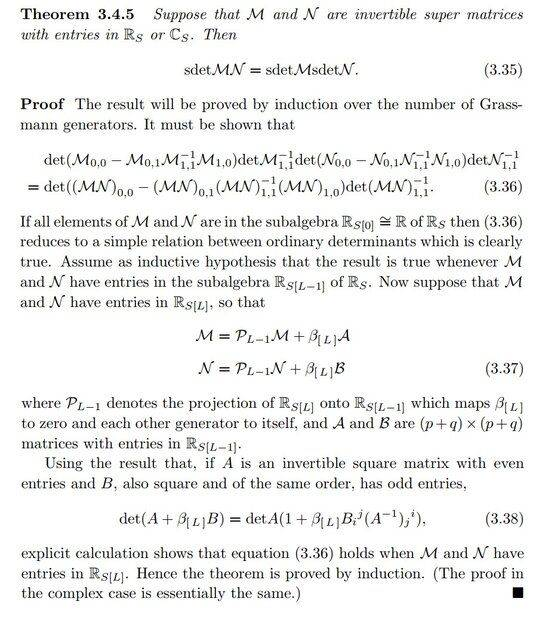# Inductive proof for multiplicative property of sdet

• B
• Korybut

#### Korybut

TL;DR Summary
Is it doable?
Hello!

Reading Roger's book on supermanifolds one can find sketch of the proof for multiplicative property of super determinant. Which looks as followsAll the words sounds reasonable however when it comes to the direct computation it turns out to be technical mess and I am about to give up. I mean all the matrices that need to be inverted are invertible and can be represented as formal power series so everything is well-defined nonetheless resulting expressions are of insane technical complexity. Have someone tried this particular way of proving this property?

To add some detail of my struggle. I represent my super matrices according to suggested in the proof way
##\mathcal{M}=\left(\begin{matrix}M_{00}+\beta_L A_{00} & M_{01}+\beta_L A_{01} \\
M_{10}+\beta_L A_{10} & M_{11}+\beta_L A_{11}\end{matrix}\right)##

##\mathcal{N}=\left(\begin{matrix}N_{00}+\beta_L B_{00} & N_{01}+\beta_L B_{01} \\
N_{10}+\beta_L B_{10} & N_{11}+\beta_L B_{11}\end{matrix}\right)##
Since it is clear that result will be only linear in ##A## or ##B## I will provide only the part that comes with ##A_{01}## (nothing particular special about ##A_{01}##. I just want to see what comes here). After straight-forward computation one has
##sdet\mathcal{M}\, sdet \mathcal{N}=sdet M\, sdet N\, \left(1-\beta_L A_{01}M_{11}^{-1} M_{10} \left(M_{00}-M_{01} M_{11}^{-1} M_{10}\right)^{-1}+\ldots \right)##
I know that one should actually "take the trace" but I will omit the indices for simplicity. Appearing here matrix ##M_{00}-M_{01} M_{11}^{-1} M_{10}## is invertible since ##M_{01} M_{11}^{-1} M_{10}## has no body. Its inverse looks as follows
##\left(M_{00}-M_{01} M_{11}^{-1} M_{10}\right)^{-1}=\sum_{p=0}^\infty \left(M_{00}^{-1} M_{01} M_{11}^{-1} M_{10}\right)^p M_{00}^{-1}##
Series has only finite amount of terms so one doesn't need to worry about convergence properties.

On the other hand one can compute ##sdet \mathcal{M} \mathcal{N}## and again examine what comes with ##A_{01}##. Since resulting formula is very involved I introduce following shorthand notation
##\mathcal{X}=M_{00} N_{00}+M_{01}N_{10}-\left(M_{00}N_{01}+M_{01}N_{11}\right) \left(M_{10}N_{01}+M_{11}N_{11}\right)^{-1}\left(M_{10} N_{00}+M_{11}N_{10}\right)##
This one of the parts that comes computing ##sdet MN##.

##\mathcal{Y}=N_{10}-N_11 \left(M_{10}N_{01}+M_{11}N_{11}\right)^{-1}\left(M_{10}N_{00}+M_{11}N_{10}\right)##.
Once again all the matrices that need to inverted are invertible as a formal series.

Using these notation result for computation ##sdet \mathcal{M}\mathcal{N}## looks as follows
##sdet \mathcal{M}\mathcal{N}=sdet MN\, \left(1+\beta_L A_{01} \mathcal{Y} \mathcal{X}^{-1}+\ldots \right)##

I've tried to show equality order by order in ##N_{01}##. It is not that hard to show that in zeroth order (i.e. ##N_{01}=0##) equality holds. However when it comes to first order it turns out to be too involved.